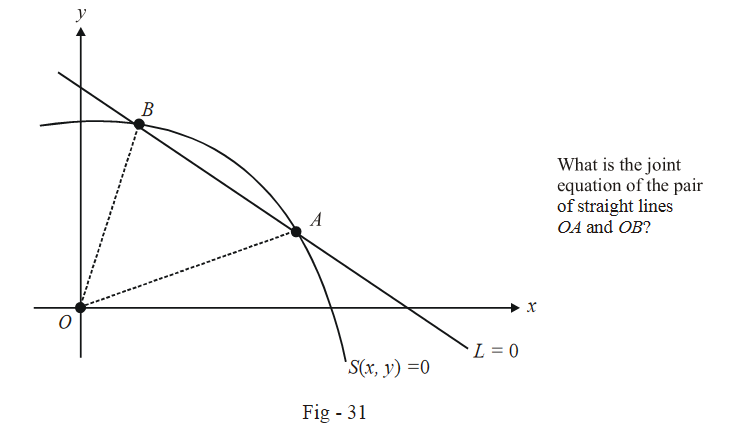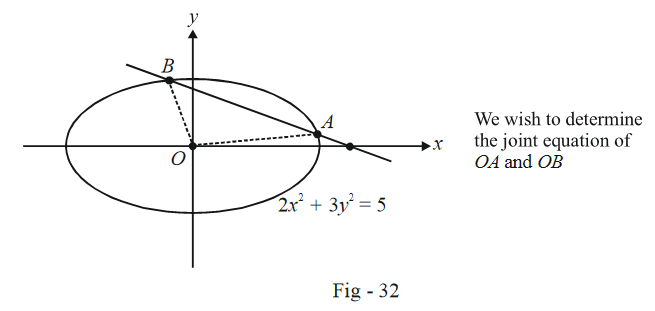# Technique of Homogenizing

We now discuss a very useful application of the concept of pair of straight lines.

Consider a second degree curve $$S(x,\;y)$$ with the equation

$S(x,\;y) \equiv a{x^2} + 2hxy + b{y^2} + 2gx + 2fy + c = 0$

and a straight line

$L \equiv px + qy + r = 0$

intersecting $$S = 0$$ in A and B. Let O be the origin.

What is the joint equation of OA and OB?The insight that we use here is that since both OA and OB pass through the origin, their joint equation will be homogenous. We now construct a homogenous equation and show that both A and B satisfy it; that equation is then guaranteed to jointly represent OA and OB.

First of all, observe that since A and B satisfy the equation of L, i.e. $$px + qy + r = 0,$$ they will also satisfy the relation

\begin{align}\frac{{px + qy}}{{ - r}} = 1 \qquad \quad : \qquad \quad {\text{Both A and B will satisfy this relation.}}\end{align}

Now, we homogenize the equation of the second degree curve $$S(x,\;y)$$ using the relation above; consider this equation :

\begin{align}a{x^2} + 2hxy + b{y^2} + 2gx\left( {\frac{{px + qy}}{{ - r}}} \right) + 2fy\left( {\frac{{px + qy}}{{ - r}}} \right) + c{\left( {\frac{{px + qy}}{{ - r}}} \right)^2} = 0 \qquad \qquad \qquad ...(1)\end{align}

Can you understand why we’ve done this? The equation we obtain above is a second degree homogenous equation, and so it must represent two straight lines passing through the origin. Which two straight lines? Since A and B satisfy the equation of the original curve as well as the relation \begin{align}\frac{{px + qy}}{{ - r}} = 1,\end{align} A and B both satisfy the homogenized equation in (1).

What does this imply ? That (1) is the joint equation of OA and OB!

Go over this discussion again if you find this confusing. You must fully understand the described technique which will find very wide usage in subsequent chapters.

Example - 24

Find the joint equation of the straight lines passing through the origin O and the points of intersection of the line $$3x + 4y - 5 = 0$$ and the curve $$2{x^2} + 3{y^2} = 5.$$

Solution: One approach is of course to explicitly determine the two points of intersection, say A and B, writing the equations of OA and OB, thereby obtaining the required joint equation. You are urged to do this as an exercise.

However, we’ll use the homogenizing technique just described:The required equation can be obtained by first writing the line as

$\left( {\frac{{3x + 4y}}{5}} \right) = 1$

and then using this relation to homogenize the equation of the curve:

\begin{align} &2{x^2} + 3{y^2} = 5{\left( {\frac{{3x + 4y}}{5}} \right)^2}\\ \Rightarrow \qquad & 10{x^2} + 15{y^2} = 9{x^2} + 16{y^2} + 24xy\\ \Rightarrow \qquad & {x^2} - 24xy - {y^2} = 0 \end{align}

This is the required joint equation of OA and OB.

Example - 25

Find the value of $$m,$$ if the lines joining the origin O to the points of intersection A, B of $$y = 1 + mx{\text{ and }}{x^2} + {y^2} = 1$$ are perpendicular.

Solution: The joint equation of OA and OB is

\begin{align} &{x^2} + {y^2} = {(y - mx)^2}\\ \Rightarrow \qquad & (1 - {m^2}){x^2} + 2mxy = 0 \qquad \qquad \qquad ...(1) \end{align}

The condition for perpendicularity is

$a+b=0$

which when applied to (1) yields

\begin{align} &1 - {m^2} = 0\\ &m = \pm 1 \end{align}

This example was more or less trivial and a little knowledge of circles would have enabled you to solve this question without resorting to the homogenizing approach; however, the fact that this approach is very powerful in many cases will become apparent in later examples.

# TRY YOURSELF - II

Q1.  Find the values(s) of m for which the following equation(s) represents a pair of straight lines:

(a)  $$x^2 + \lambda xy - 2y^2 + 3y - 1 = 0$$

(b)  $$4x^2 + 10xy + \lambda y^2 + 5x + 10y = 0$$

Q2.  Find the angle of intersection of the straight lines given by the equation

$$3x^2 - 7xy + 2y^2 + 9x + 2y - 12 = 0$$

Q3.  Show that the lines joining the origin to the points common to $$x^2 + hxy - y^2 + gx + fy = 0$$ and $$fx - gy = \lambda$$ are at right angles for all values of $$\lambda.$$

Q4.  Find the angle between the lines given by $$x^2 - 2pxy + y^2 = 0$$.

Q5.  Prove that the lines joining the origin to the points of intersection of the line \begin{align}\frac{x}{h} + \frac{y}{k} \ne 2\end{align} with the curve $$(x - h)^2 + (y - k)^2 = c^2$$, are perpendicular if $$h^2 + k^2 = c^2$$.

Q6.  Find the joint equations of the straight lines passing through (1, 1) and parallel to the lines given by $$x^2 - 5xy + 4y^2 + x+2y - 2 = 0$$.

Q7.  Evaluate the point of intersection for the lines represented by the general equation $$ax^2 + 2hxy + by^2 + 2gx + 2fy + c = 0$$.

Q8.  Find the joint equation of the images of the pair of lines $$ax^2 + 2hxy + by^2 = 0$$ in the mirror $$y = 0$$.

Q9.  Find the joint equation of the angle bisectors of the lines given by $$x^2 + 2xy\sec \theta + y^2 = 0$$.

Q10.  If the pairs of straight lines

\begin{align} & ax^2 + 2hxy + by^2 = 0 \\ &a'x^2 + 2h'xy + b'y^2 = 0 \end{align}

have a line in common, show that

\begin{align}\left( {\frac{{ab'-a'b}}{2}}\right)^2=(ha'-h'a)(h'b-hb')\end{align}